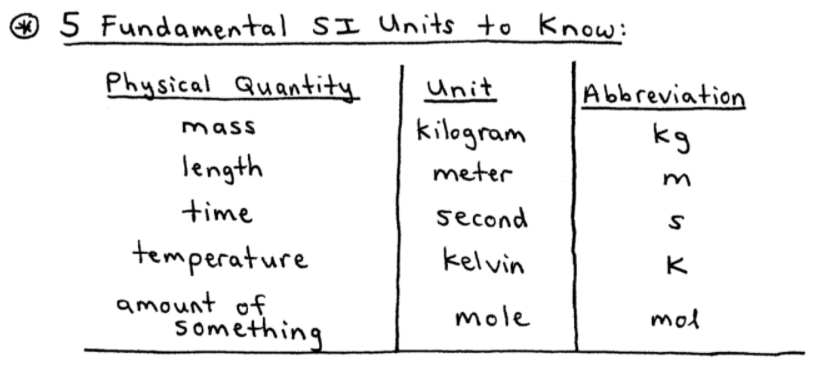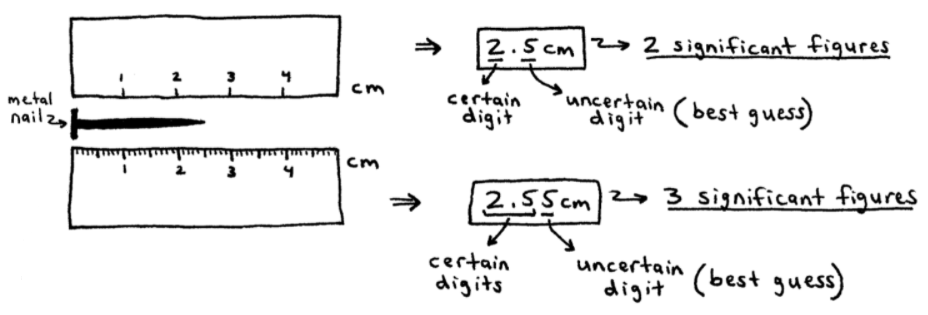# S1E2 - SI Base Units, SI Prefixes, & Units of Measurement

Measurements are quantitative observations that consist of two parts:

• number  ( ex: 4 )
• unit  ( ex: grams )

➞ combined, this gives  =  4g

==========

## Units of Measurement

For measurements in different parts of the world to be comparable and meaningful, a standard system was adopted:

## SI System of Units

The SI stands for the French way of saying "International System of Units."

While there are a total of 7 SI Base Units, the five SI Base Units you should learn are listed below...

==========

## SI Base Units

SI Base Units are also called "SI Fundamental Units" and are shown below:There are actually 7 SI Base Units, but electric current (ampere) and luminous intensity (candela) are not shown above.

The five SI Base Units you see above?

Learn these!

==========

## SI Derived Units

All other units are SI Derived Units because they're derived from the 7 SI Base Units.

Common examples include:

➞ density
➞ volumeThe SI Base Units (Fundamental SI Units) and SI Derived Units are not always useful, so prefixes are often used...

==========

## SI Prefixes

In the image below, we've listed some of the most common SI Prefixes.

You should learn these!...==========

## Mass vs. Weight

We won't spend too much time on this, but "mass" and "weight" are NOT interchangeable terms.

Check out the definitions of each below...

----------

Mass  =  measure of the amount of matter in an object.

Matter, in turn, is anything that has mass and takes up space.

Weight  =  the force that gravity exerts on an object.

----------

ex:  An astronaut has the same mass on Earth as he does on the Moon, but he weighs about seven times less on the Moon due to a smaller gravitational pull.

==========

## Uncertainty in Measurement

Every measurement has some degree of uncertainty associated with it.

The uncertainty depends on the precision of the measurement.

ex:  Do the two grapefruits weigh the same?..._________

➞  Both YES and NO.

WHY?

Because it depends on the precision of the measuring device being used.

➞ If using a bathroom scale YES they weigh the same.

➞ If using an analytical balance ➞ NO they do not weigh the same.

==========

ex:  Which measurement below has the most uncertainty?

Which measurement is the most precise?_________

➞  The second measurement in the above sketch (2.55 cm) is the most precise and thus has the least amount of uncertainty associated with it.

----------

So... the greater the amount of significant figures there are, the lesser the amount of uncertainty.

A measurement's uncertainty is expressed by the number of significant figures (sig. figs).

Okay that's great.

But what the heck are significant figures... ?

==========

## Significant Figures in Measurement

Significant Figures  =  the number of significant figures in a measurement is equal to the number of certain digits and one uncertain digit.

The greater the number of sig. figs., the lower the degree of uncertainty.

The greater the number of sig. figs., the more precise the measurement is.

==========

Next up, we'll continue to focus on SECTION 1 - Foundations of Chemistry, where we'll discuss the:

Two Types of Errors in Measurement, and Accuracy vs. Precision.